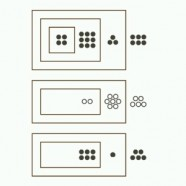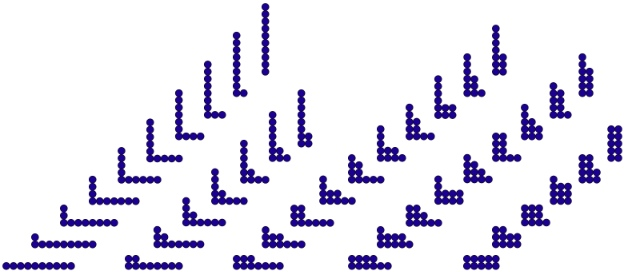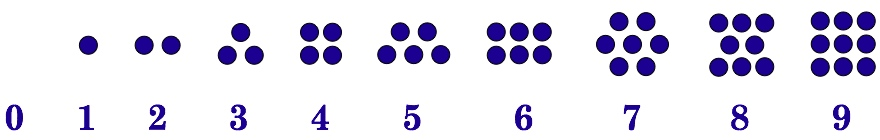# Decimal Unit CalculatorThe Iconic Arithmetic Calculator set to decimal unit mode shows computation using our conventional base-10 numbers with two modifications. Depth-value replaces place-value and unit-ensembles replace digits. Depth-value removes the linear sequential restrictions of place-value. Unit-ensembles remove the need to memorize digit number facts. The Image shows three decimal numbers about to be added, one is negative. Positive units are indicated by full circles, , while negative units are indicated by empty circles, . Decimal unit numbers are particularly convenient for showing how our number system works, and in particular how addition works.  When ten units share the same container, they are grouped together as a single unit in a new container. We interpret the new container, (●), as multiplying its contents by ten. Using iconic units rather than symbolic digits frees us from having to memorize both the meaning of the digit symbols and the results of their additive combinations (the addition table). Other than grouping size, the rules of iconic arithmetic are the same in all bases. What defines a decimal number is that ten of them make a group.

The addition shown in the image, 2936+(–577)+518, is animated below as an introduction. For context, the entire sequence of entering these numbers into the Iconic Calculator is included for this first example. The rest of the page then shows the Calculator display window only for many other animations that illustrate the details of how iconic decimal addition with unit-ensembles works.

The decimal unit iconic calculator: 4936+(–275)+615=5276

## Unit Ensembles rather than Digits

Symbolic digits encode a group of units. The meaning of the symbolic digit is how many units the digit is intended to stand in place of. For example, 4 stands in place of ●●●●. Digits are symbolic, they share the symbolic characteristics of being meaningless in themselves, and requiring memorization to know their assigned meaning. Unit ensembles are iconic, a group of units looks like how many that group represents. The Decimal Unit Calculator shows the meaning of digits directly, thus eliminating the symbolic abstraction barrier that separates digits from reality itself while requiring memorization of their meaning.

There are 96 possible ways to put groups to digits together so that they add up to less than ten. Unit ensembles count for themselves; that is, we do not need to count them to know how many there are, we can use to ensemble itself as the counting mechanism. The 96 ways units add to less than ten reduces to the 8 different piles that arise when units are put into the same container. In an iconic system, there is not representation of 0, and it is not possible that 1 is the result of any addition. That leaves the eight groups from 2 to 9 as sums.

There are 42 different ways that digits can add to exactly ten, illustrated below using units rather than digits. For each connected cluster, read the height of a stack as the value of the digit and the number of columns as how many digits are being added. The leftmost cluster is ten 1s being added. The rightmost cluster is 5+5.Forty-two ways to group units to add to ten

The illustration shows units rather than numbers in order to illustrate the standardization of unit ensembles. Read the height of a stack as an ensemble of units in one container and the columns as different containers to be Merged. The leftmost cluster presumes that each unit begins as separated from the rest. The Group rule would convert these ten separate units into one base-10 Group, (●). The rightmost cluster presumes that we begin with two containers, each containing “5” units. But iconic addition is defined without regard to the contents of each container. Addition is achieved by putting containers into the same outer container. Except for the magnitude of the eventual ensemble, there is nothing numerical that differentiates any of the 42 clusters. Once the process of addition has been initiated, how many containers are being Merged and how many units are in each of those containers are details of the steps in the standardization procedure, not essential aspects of adding. Iconic arithmetic provides students with a clear distinction between concept and manipulation. Symbolic arithmetic requires counting units to achieve addition, rather than allowing units to stand for themselves. Of course, symbolic digits cannot stand for themselves, they are codes not concepts. That is, each of the 42 configurations is 10. Fusion maintains no memory of how an ensemble has been constituted. The 42 different symbolic addition facts reduce to one iconic counting of the resulting ensemble.

## Iconic Addition with Depth-Value

The addition process consists of putting groups of units together. Both place-value and depth-value require further steps to achieve a sum. Place-value has the well-known steps of aligning digits in columns and “carrying” digit overflow into adjacent columns on the left. The nested containers of depth-value automatically take care of alignment of magnitudes, while carrying is partitioned into the Group and Merge actions.

### Grouping Units

Grouping joins ten units together in one container that is interpreted as multiplying its contents by ten.

Grouping ten units: 7+3=10

When less than ten units are put together, no further action is necessary. For ease of reading only, units are clustered into characteristic patterns for magnitudes from 2 to 9. The unit number patterns used by the Unit Calculator are shown below.Merging combines the contents of all containers within an outer container. This video shows four containers merging into one. Since the contents of the four containers add to less than ten, no Grouping occurs.

Merging boundaries: 2+1+3+2=8

Canceling deletes pairs of units with opposite polarity. This video show 1 and –1 canceling, leaving a void space.

Canceling units of opposite polarity: 1+(–1)=0

## Exploring Decimal Unit Addition

Depth-value provides the opportunity for parallel actions in each separately nested space. Should a collection of units at any level of nesting be equal to less than ten no further action is necessary, although units are moved together for ease of reading. Should units at a particular level sum to ten or more, then those units are grouped. The first video shows simple addition by Merging boundaries and no resulting grouping.

Merging boundaries: 24+31+13=68

The next video shows addition via Merging boundaries while one level of nesting adds to more than ten. Ten units are grouped and the resulting boundary merged with the next deeper level.

Merging boundaries with Grouping: 24+35+13=72

This video shows addition via Merging boundaries with units at several levels of nesting adding to more than ten. All grouping occurs in parallel.

Several levels Grouping: 8256+3375=11631

This video shows addition via Merging boundaries with units at one level of nesting adding to more than thirty. Three groups are formed and merged with the next inner level.

Parallel Grouping: 182+193+290+282=947

This video shows addition via Merging boundaries. Many concurrent groups of ten are created.

Lots of parallel grouping: 7777+9898+8768+8989=35432

When newly formed groups are Merged with the next level of nesting, the additional unit(s) at that deeper level may themselves add to more than ten. This propagates a sequence of Grouping followed by Merging. This video shows a sequence of grouping and merging.

Propagation of grouping: 3063+1980=4143

Sometimes the sequence of grouping following by Merging can propagate inward over several levels. Here is an example.

Sequential propagation of grouping: 98765+1234+1=100000

Finally, an example with all addition dynamics occurring at the same time.

## Decimal Unit Subtraction

The concept of subtraction is subsumed by addition of negative numbers which are represented by hollow circles, . Cancelation of opposite polarity units within each level of a depth-value number may leave different levels with different polarity. Container numbers support a form foreign to conventional numbers, mixed polarity numbers. Mixed polarity is an intermediate form, prior to complete standardization of a number into a form with one single polarity, conventionally a number that is either positive or negative. When positive and negative numbers are added together, the first action is to cancel all pairs of opposite polarity. For visual convenience the Decimal Unit Calculator identifies units to be matched, superimposes positive and negative units, and deletes both by fading into nothing. Here is an example.

Subtraction via Canceling: 8–2=6

In contrast to digit subtraction shown on the Decimal Digit Calculator page, it is not necessary to Ungroup units in decimal unit computation since units are already ungrouped. Spatial localization of units is visual but not computational since there is no meaning associated with where units are within a given nested layer. A fundamental concept for iconic representation is that empty space has no properties. All that is needed is to match units one-to-one with units of opposite polarity. Here is a more substantive example of pairing opposite units.

Canceling by matching opposite units: 9–1+9–7–4–6–6+9=3

Nor is it necessary to identify the optimal patterns to Cancel. In this example, some opposite units are Canceled, leaving others to be Canceled later, when the available pairs are more easily identified.

Canceling in successive passes: –8+6+4+6–5=3

Here is an example of canceling occurring at every nested level.

Canceling at many levels: 47906–55725+97353=89534

### Mixed Polarity Numbers

Each level of nesting contains an independent sum of units. When positive and negative units are added together, different levels may Cancel to reach different polarities. These mixed polarity numbers are intermediate stages in the standardization process. Next are examples that show the elimination of mixed polarity. The polarity of the unit(s) at the deepest level of the sum determines the polarity of the standardized number. Opposite units in shallower levels are canceled by migrating one unit of the dominant polarity outward. Moving units outwards consists of first unmerging one unit from a deeper level and then ungrouping it at the next shallower level to a ensemble of ten. We can call this sequence of unmerging one unit and then ungrouping it, unmixing. The next video illustrates a simple case of unmixing.

A simple case of Unmixing: 34–8=26

Cancellation of outer units is achieved by the opposite unit in the nearest deeper level. In this example, after canceling, three separate levels Unmerge concurrently.

Unmerging on three levels concurrently: 4467362–562805=3904557

When a unit of opposite polarity is separated by empty levels, the unmerged unit must migrate across these empty levels. Here is an extreme example.

Unmerging across many empty levels: –654321+54328=599993

In this example, unmerging occurs in a sequence from the deepest level to the shallowest level.

Sequential unmerging across many levels: 400000–24568=375432

Here units unmerge concurrently to encounter several empty levels.

Concurrent ungrouping across empty levels: TO DO

It is generally better to group units of either type before unmerging to cancel mixed levels. In this example the many negative units in shallower levels group and merge first prior to being cancelled by the innermost positive units.

Merging prior to canceling: 122–59+301–98–98=178

In the next example, performing the Group operation first eliminates any need to later Unmerge.

Merging prior to canceling: –725–919+70–507+2080=–1

This next example illustrates that processes at each nesting level are conceptually independent. After the initial canceling, the innermost positive units and the next level’s negative units both group, even though the innermost positive group is later ungrouped in order to cancel outer units. It is preferable to localize rules and then perhaps reverse some grouping than to attempt to take into account activities at different levels. In conventional subtraction of place-value numerals, a student must either subdivide the task into multiple problems (here perhaps adding all the positive units and then adding all the negative units prior to doing the final subtraction), or alternatively look ahead across several place-value columns. The simplicity of parallelism more than compensates for an occasional reversal of a grouping.

Grouping first without planning: 752–81+311–95=887

[19, 18, 18, -70, 17, 17, -91, 5]

Concurrent ungrouping across empty levels: TO DO